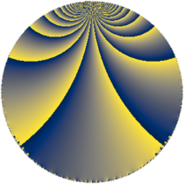# Properties

 Label 224.4.uLevel $224$ Weight $4$ Character orbit 224.u Rep. character $\chi_{224}(29,\cdot)$ Character field $\Q(\zeta_{8})$ Dimension $288$ Newform subspaces $2$ Sturm bound $128$ Trace bound $1$

# Related objects

## Defining parameters

 Level: $$N$$ $$=$$ $$224 = 2^{5} \cdot 7$$ Weight: $$k$$ $$=$$ $$4$$ Character orbit: $$[\chi]$$ $$=$$ 224.u (of order $$8$$ and degree $$4$$) Character conductor: $$\operatorname{cond}(\chi)$$ $$=$$ $$32$$ Character field: $$\Q(\zeta_{8})$$ Newform subspaces: $$2$$ Sturm bound: $$128$$ Trace bound: $$1$$ Distinguishing $$T_p$$: $$3$$

## Dimensions

The following table gives the dimensions of various subspaces of $$M_{4}(224, [\chi])$$.

Total New Old
Modular forms 392 288 104
Cusp forms 376 288 88
Eisenstein series 16 0 16

## Trace form

 $$288q + O(q^{10})$$ $$288q - 240q^{10} + 96q^{12} + 300q^{16} + 180q^{18} - 628q^{22} - 328q^{23} - 912q^{24} - 40q^{26} + 528q^{27} - 1160q^{30} - 1240q^{32} - 1000q^{34} - 2480q^{36} - 2408q^{38} - 1200q^{39} + 1640q^{40} - 808q^{43} + 500q^{44} + 2880q^{46} + 4888q^{48} + 4264q^{50} + 2976q^{51} + 1728q^{52} - 752q^{53} + 1728q^{54} + 576q^{55} - 196q^{56} - 2376q^{58} - 8552q^{60} + 3648q^{61} - 6840q^{62} - 2520q^{63} - 6592q^{66} - 2040q^{67} - 5032q^{68} + 2112q^{69} - 1008q^{70} + 3408q^{72} + 1316q^{74} + 4416q^{75} + 10240q^{76} - 1904q^{77} + 5064q^{78} + 15600q^{80} + 5840q^{82} + 1848q^{86} + 2576q^{87} - 3120q^{88} - 3720q^{90} - 13692q^{92} - 16264q^{94} + 12160q^{95} - 12352q^{96} + 10624q^{99} + O(q^{100})$$

## Decomposition of $$S_{4}^{\mathrm{new}}(224, [\chi])$$ into newform subspaces

Label Dim. $$A$$ Field CM Traces $q$-expansion
$$a_2$$ $$a_3$$ $$a_5$$ $$a_7$$
224.4.u.a $$140$$ $$13.216$$ None $$0$$ $$0$$ $$0$$ $$0$$
224.4.u.b $$148$$ $$13.216$$ None $$0$$ $$0$$ $$0$$ $$0$$

## Decomposition of $$S_{4}^{\mathrm{old}}(224, [\chi])$$ into lower level spaces

$$S_{4}^{\mathrm{old}}(224, [\chi]) \cong$$ $$S_{4}^{\mathrm{new}}(32, [\chi])$$$$^{\oplus 2}$$